# Using Google BigQuery As A Massively Parallel Scientific Calculator: From π to Monte Carlo

An idea was discussed a bit at a talk I gave on BigQuery at a Google Developer Group meetup in Chattanooga. Can you use BigQuery to generate massive data instead of simply loading or analyzing existing data? Sure. One can write up a giant `select` query to fix some errors in a dataset, rearrange the column order, calculate some new columns, and save it in a table.
But something more basic, even subversive, is possible. It could be a Hadoop killer for some calculations.
All we need is a table of integers, say a list from 1 to 1,000,000,000.
Not finding such a dataset we release one as `eaftc-free:billion.integers` for public exploration.
Although this dataset would seem to be uninteresting, even trivial, it allows the end user to build all sorts of calculations and simulations using mathematical functions and the uniform `[0,1)` random function `rand()` built into BigQuery.
Processing costs are your own responsibility, but Google has a limited free tier. Clicking this link will take you to a Google BigQuery signup unless you already have a BigQuery account.
So what? You ask. This simple table was a missing piece to make a massive parallel calculator.

# Normal Usage

Normally, π in Google BigQuery can be obtained from the built in function `PI()`
Warning: I do not necessarily advocate repetition of the pi calculations shown below. You should use your free monthly processing in BigQuery to do something new and useful. Really. On the other hand, the public regularly uses similar levels of resources to search for furry kittens.
Queries can cost money… disclaimer: Most of the queries reported here will use 7 - 25 GB of BigQuery processing. After the free tier, in March 2015 Google BigQuery is currently priced by Google at `US\$5/TB`. So, some of the queries here could cost you `US\$0.10` every time you run them. Therefore, you should probably not attach a web page that shows furry kittens to a back end cgi script that runs these queries on demand for the public from your account. Also in this article are instructions for constucting large tables. These large tables also attract storage costs from Google BigQuery until you delete them. Neither the author nor my company, Economic and Financial Technology Consulting LLC, will be responsible for any Google BigQuery processing, storage, or other charges incurred by experimenting with these queries.

# Brute forcing a sum

Let’s calculate π using one of those huge sums you learn in math class.

## Leibniz formula

From the Leibniz formula for Pi
(π/4) = 1 - (1/3) + (1/5) - (1/7) +(1/9) - (1/11) + …
We can write the calculation for π from the first 2,000,000,001 (two billion and one) terms in this series as this BigQuery against `[eaftc-free:billion.integers]`:

``````SELECT 4*(1+sum( (1.0/(4.0*n+1.0))-(1.0/(4.0*n-1.0)) )) as LeibnizPI FROM [eaftc-free:billion.integers];
``````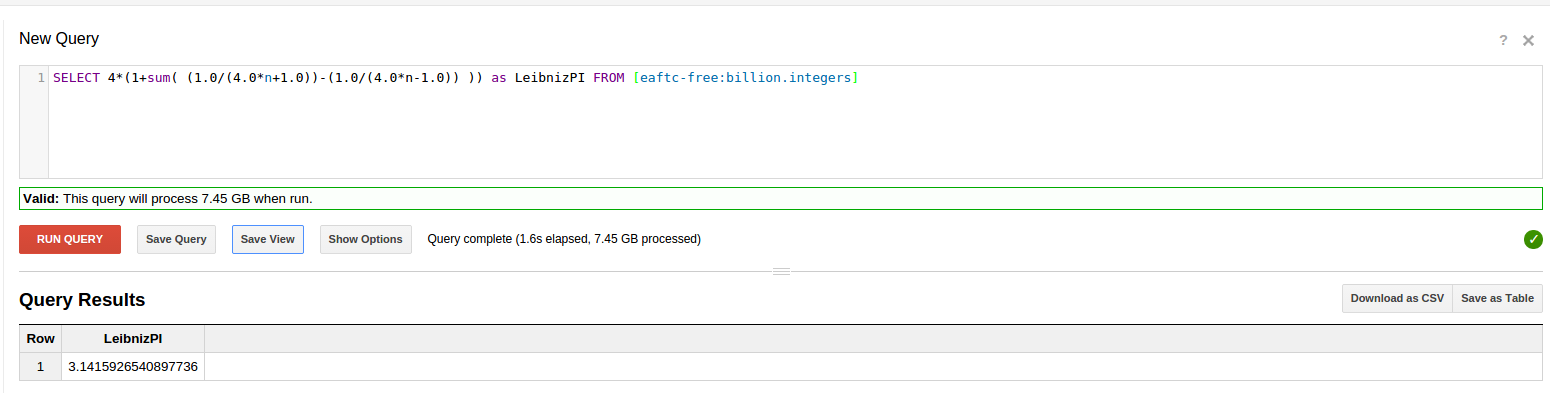Obtaining this approximation: 3.1415926540897736 in about 2 seconds
Compare to the pi day page - : 3.1415926535897932384
shows the two billion and one term Leibniz sum to be correct to 9 places and is a bit high for digits 10-11.
This sum can also be easily written as a simple loop in Python, or your favorite programming language, and is left as an exercise to the reader. A decent desktop computer, like our AMD FX8150, found a similar value in about 6 minutes running in one CPU core. Due to rounding and the kind of floats you are using, different answers are possible.

## Euler formula

Euler had a result that Pi squared over 6 is the limit of the sum of all the squared reciprocals
(1/1)+(1/4)+(1/9)+(1/16)+…
This can be written in Google BigQuery as:

``````SELECT sqrt(6.0*sum(1.0/(n*n))) as EulerPI FROM [eaftc-free:billion.integers]
``````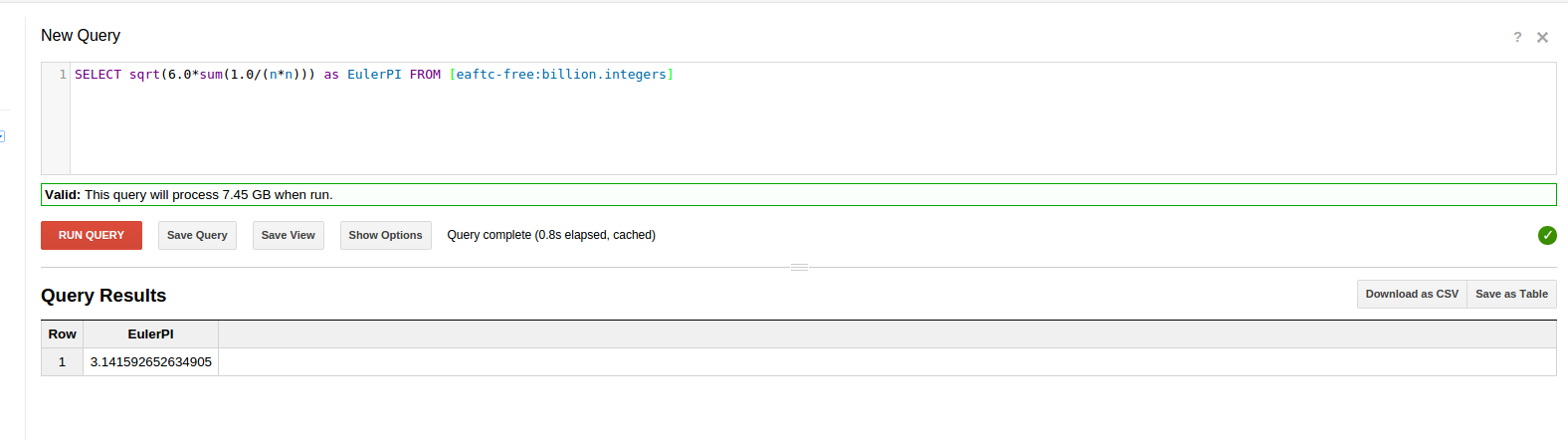Obtaining this Euler approx: 3.141592652634905
Compare to the pi day page - : 3.1415926535897932384
shows the one billion term Euler sum to also be correct to 9 places and is a bit low for digits 10-11.
Although I don’t think you should waste of all Google’s processing power recomputing π over and over, Wikipedia provides a list of approximations of π that might be reconstructed in a similar manner.

# The Road to Monte Carlo

Monte Carlo methodology has broad application in computational finance, particularly in the pricing of various kinds of bets (derivatives) about the motion of a price or price index, as well as in applied mathematics, and thus, many applications in science and engineering, to estimate integrals and dynamic processes that can not be derived on paper analytically.
The basic idea of Monte Carlo methods is:
1. Express the desired calculation as an average or other reduction of functions of a bunch of random numbers that are obtained from calling a random number generator
2. generate that bunch of random numbers
3. reduce the giant table of random numbers to the answer using other functions, averages, etc.

# Strange results repeating Big Query’s `RAND()`

You’d think multiple calls to `RAND()` in a query would generate independent calls and independent random numbers. Not so, as of March 2015.

## Should this query yield 1/4 or 1/3 of 1,000,000,000 ?

`````` select sum(rand()*rand()) from [eaftc-free:billion-integers]
``````

What this does is, sum up `rand()*rand()` 1 billion times, once for each of the integers in the table. Each call to `rand()` yields a random floating number between 0.0 and 1.0.
Notice that no columns of the table are needed, so this query attracts 0 bytes of Google BigQuery processing charges even though it is work for BigQuery to calculate. The number of rows in the billion-integers table, 1 billion, controls the number of times the innermost expression will be executed before being summed.

### Why 1/4 of 1 billion?

If the `RAND()` calls produce different, independent, random numbers, then we can use the formula that applies to independent random numbers `E[x*y]=E[x]*E[y]` where `E[x]` is the expected vaue of x, a fancy technical way to say the average of x. Since the average value of `rand()` is 0.5, we should have `avg(rand()*rand()) = avg(rand()) * avg(rand()) = 0.5 * 0.5 = 0.25`. If the avg is 0.25, then summing 1,000,000,000 should yield around 2.5E8, or 250,000,000

### Why 1/3 of 1 billion?

Cacheing is a technique to speed up computer processing by recalling a result from memory instead of processing it over again. If multiple calls to `RAND()` in a row of output were somehow cached into one call to `RAND()` then `avg(rand()*rand())` is not multiplying two different independent random numbers and taking the average, but actually multiplying a single random number by itself and taking the average, as in`avg(square(rand()))`. In such a case, the result is the definite integral of x^2 over the interval [0,1], which you might remember from freshman calculus is 1/3.

### And the Google BigQuery Answer is …. 1/3 … ugh :-(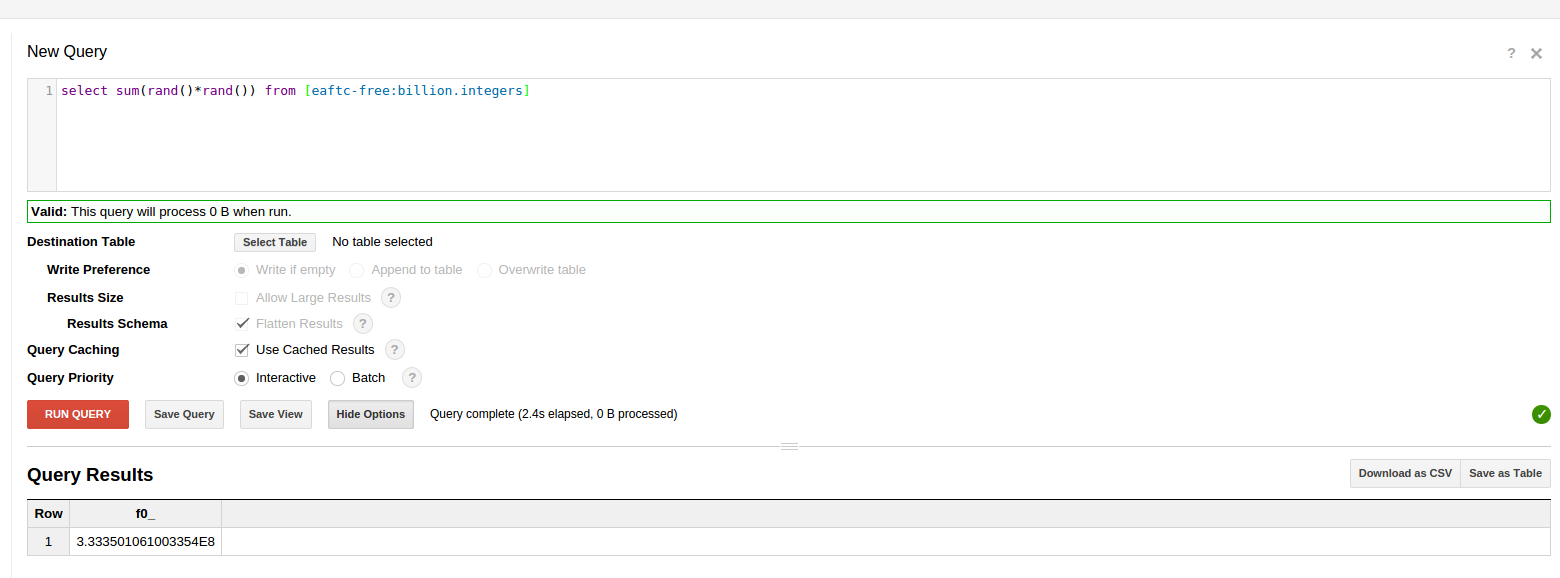# Subselects won’t generate independent tables of RAND()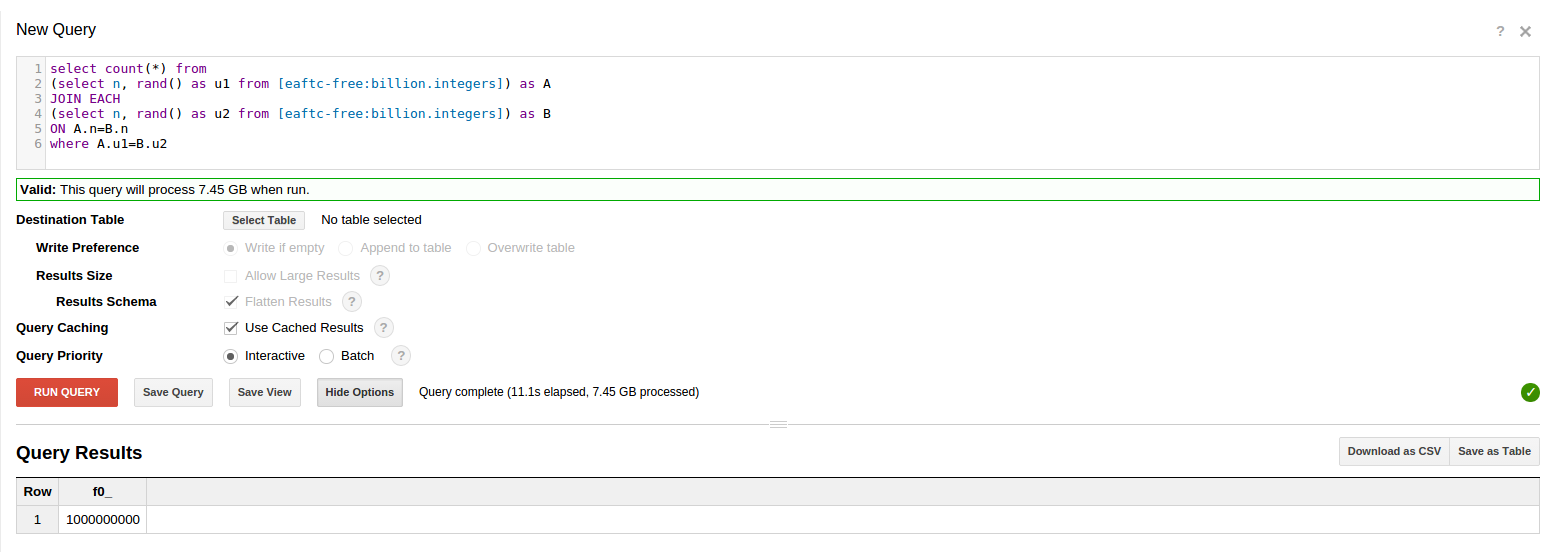The next section explores how to work around this issue to generate a table with two independent random values.

# Paving the Road to Monte Carlo

We want to generate a table with independent realizations of two random variables on each row.
Let’s call these variables `u` and `v`
This can be done in a straightforward series of steps.

1. Make a u table where u is generated by `rand()`
2. Make a v table where v is generated by `rand()`
3. Join the u and v tables
4. Test for independence
5. Clean up by deleting the tables in steps 1 and 2

We publicly released the resulting u,v table as [eaftc-free:billion.randUV]

# Making the randU table

In a query returning a billion entries into a new table, we had to select `Allow Large Results`. As a matter of courtesy to other users we also ran these table construction queries as batch mode queries.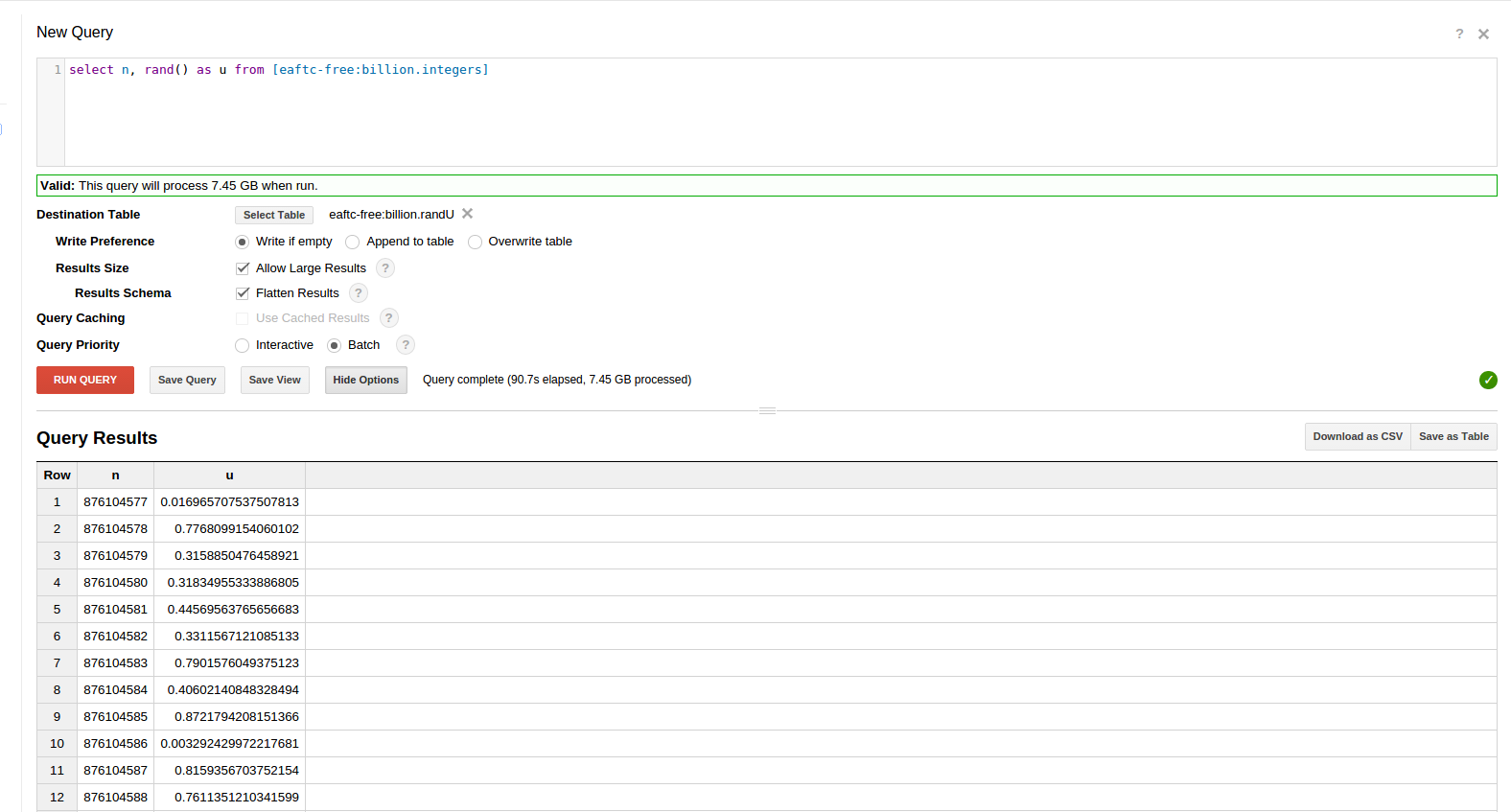# Making the randV table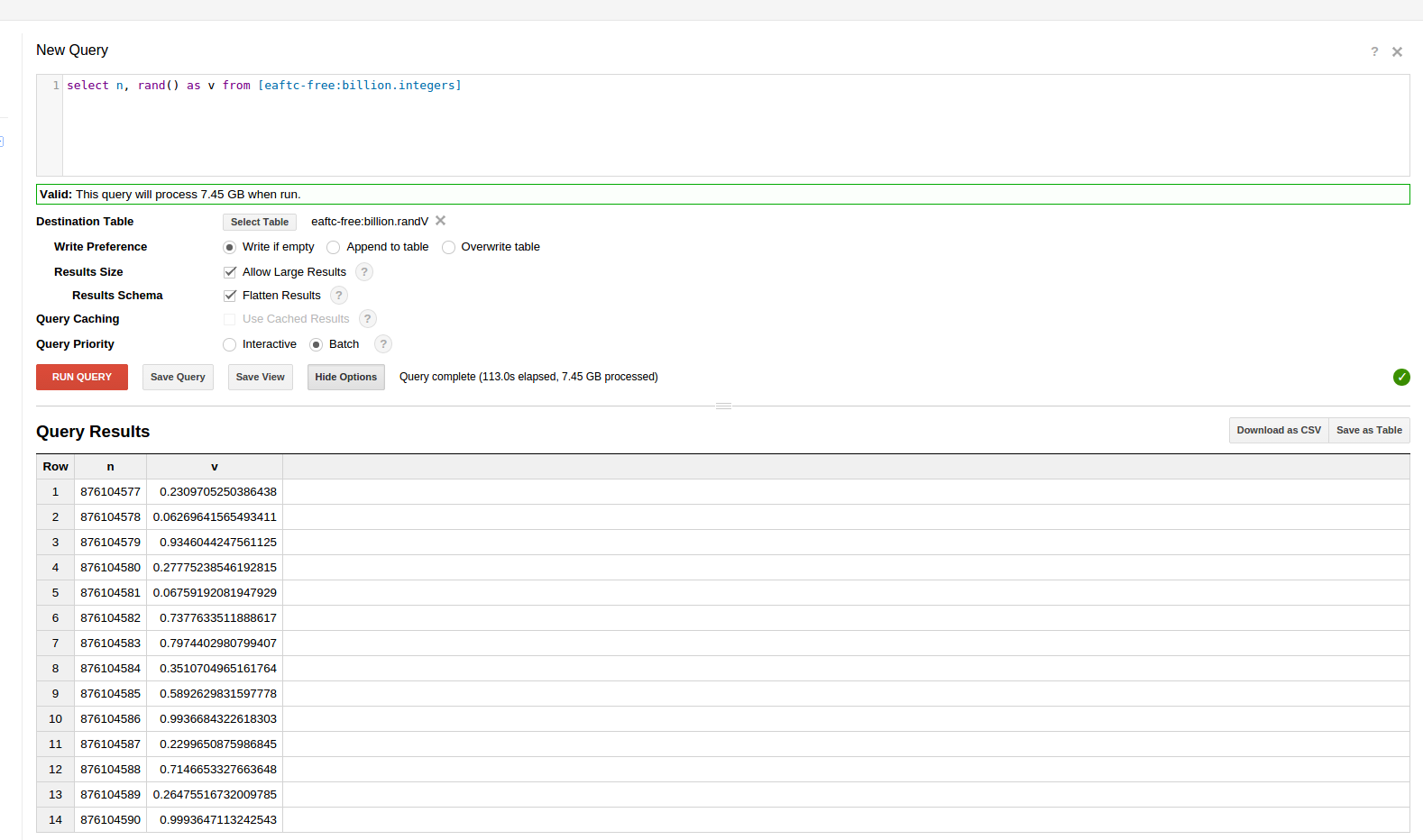# Joining to make the randUV table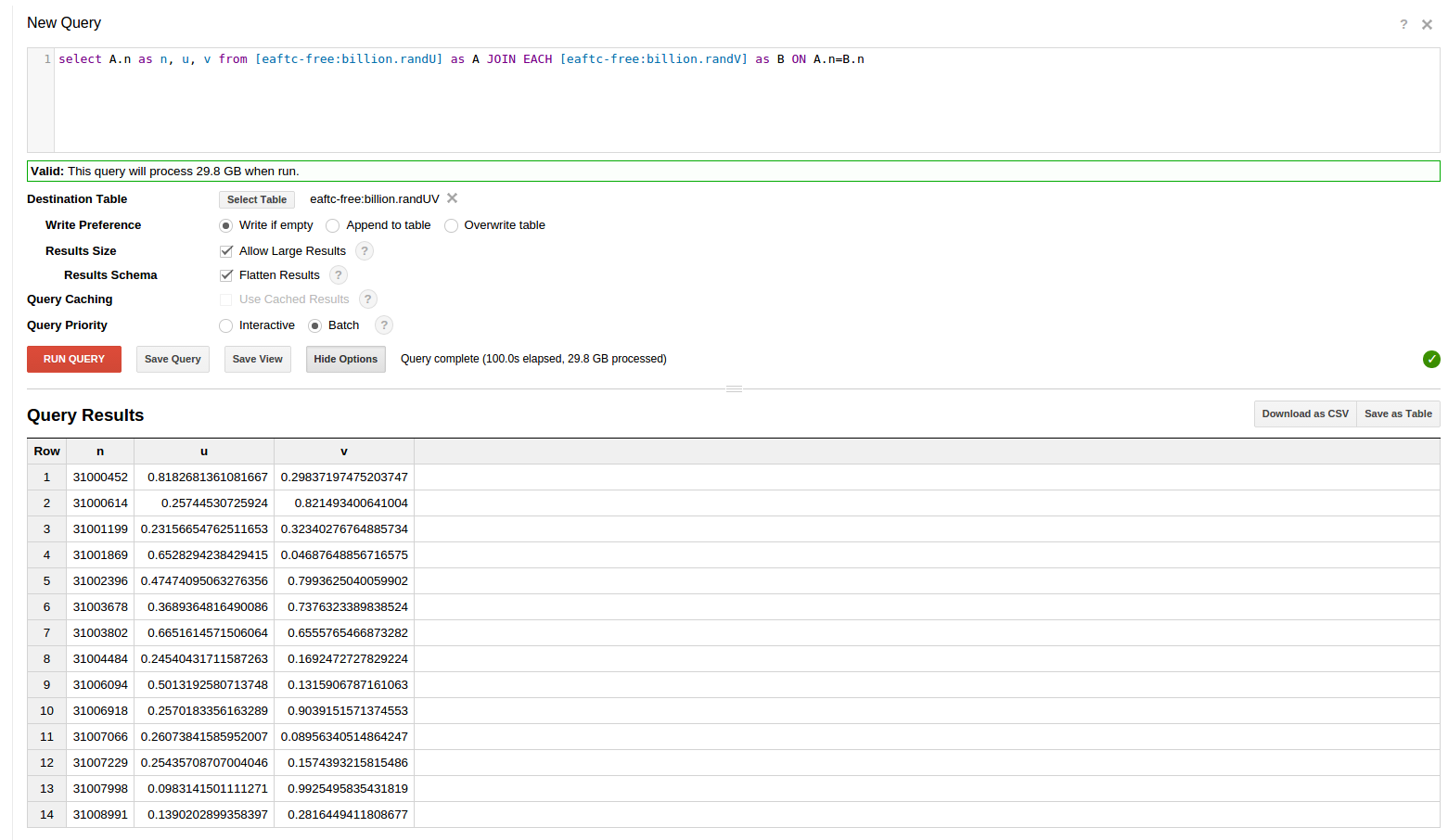# Testing independence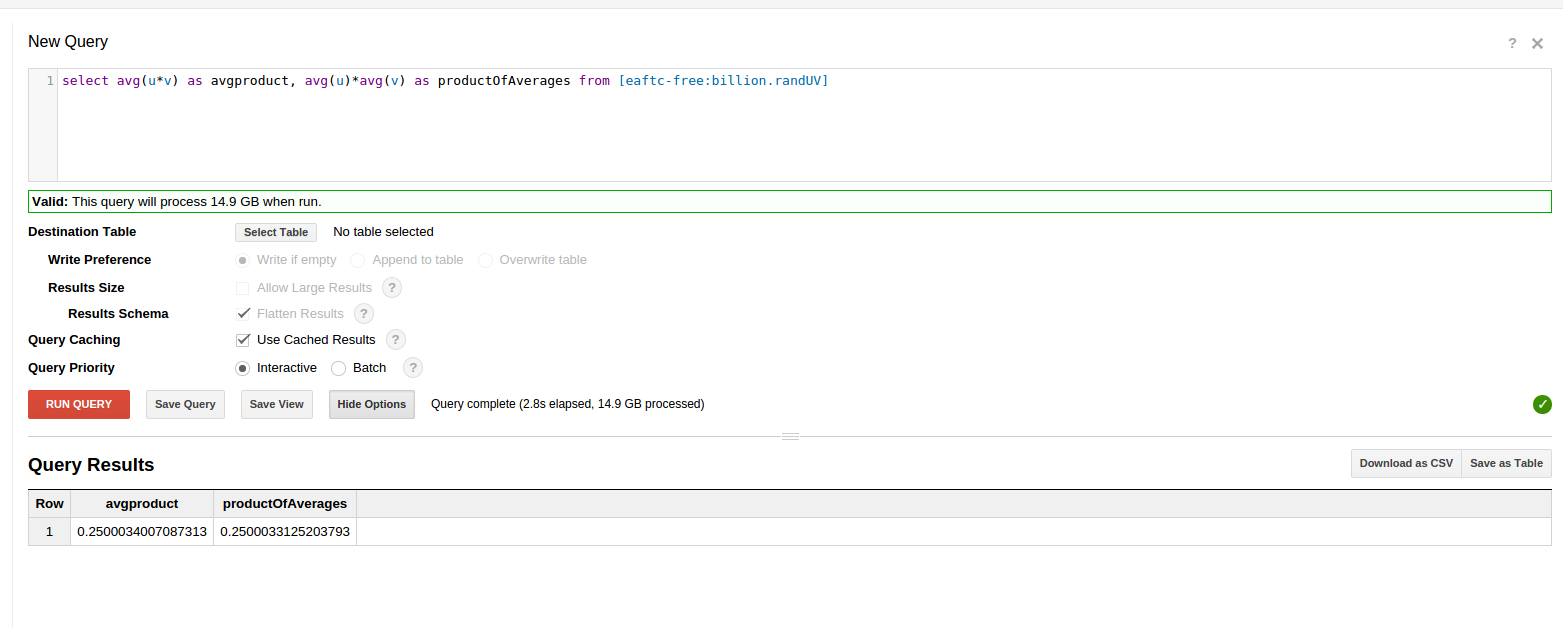# Generating Normals: The Box-Muller Transform

Having a table with two independent random uniform variables allows us to make standardized normal random variables from the normal, or bell-shape, distribution. Although we won’t get that far in this article, the normal distribution is the basic building block for building random walks of stock market prices and Monte Carlo estimation of options prices.
The Box-Muller Transform says we can take our two independent `rand()` uniform random numbers `u` and `v` and make two zero mean, unit variance standard normal distribution random numbers `z0` and `z1`using these formulas:

``````z0 = sqrt(-2.0 ln(u)) cos(2πv)

z1 = sqrt(-2.0 ln(u)) sin(2πv)
``````

In Google BigQuery, to avoid extra storage charges we store a view computing z0, z1 from u, v based on table randUV instead of computing a new table of z0, z1.
So you can follow along, we’ve made this view publicly available as `[eaftc-free:billion.BoxMuller]`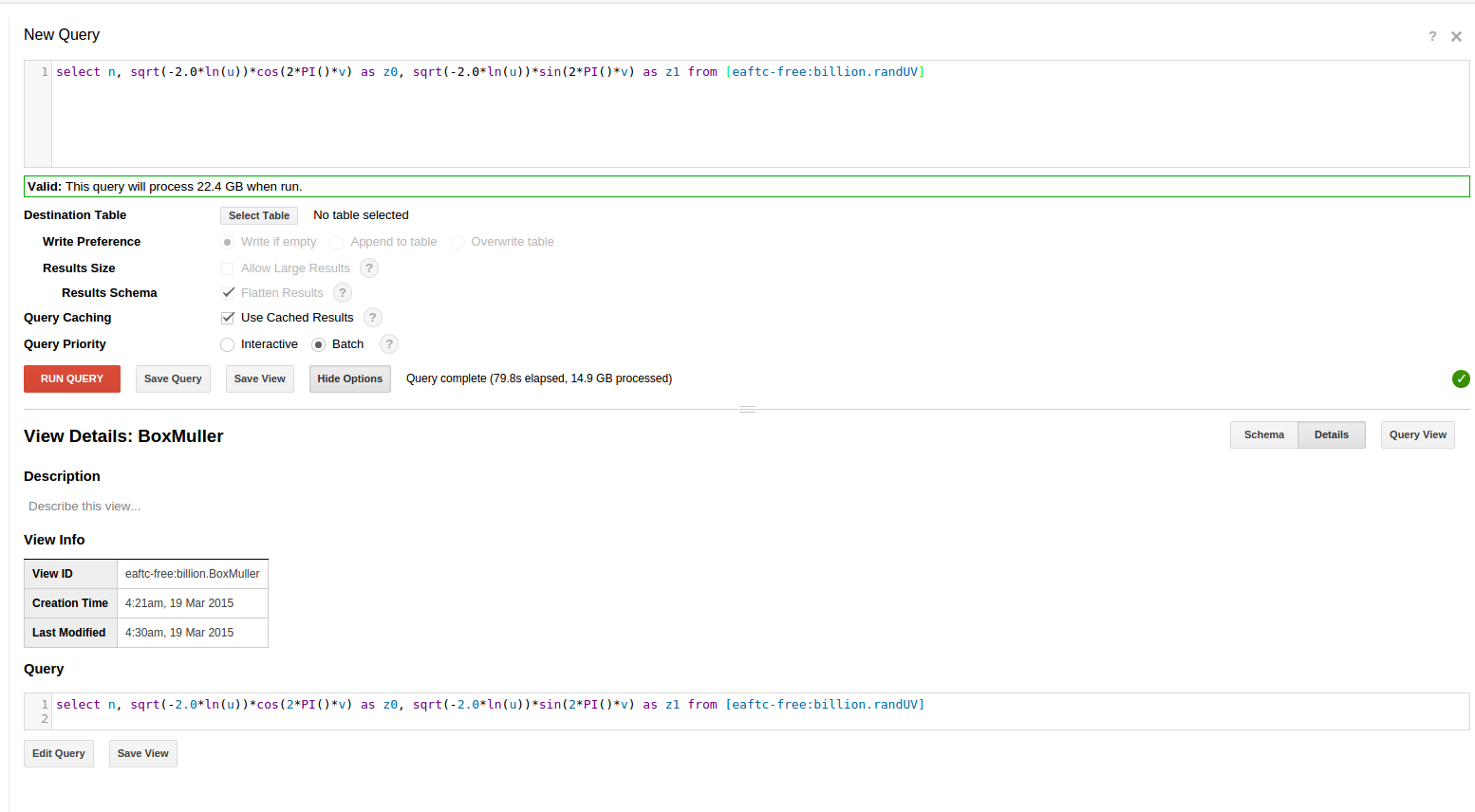# Testing averages, variances from Box-Muller

We were happy to see averages near zero and variances near 1, and all slightly different from each other.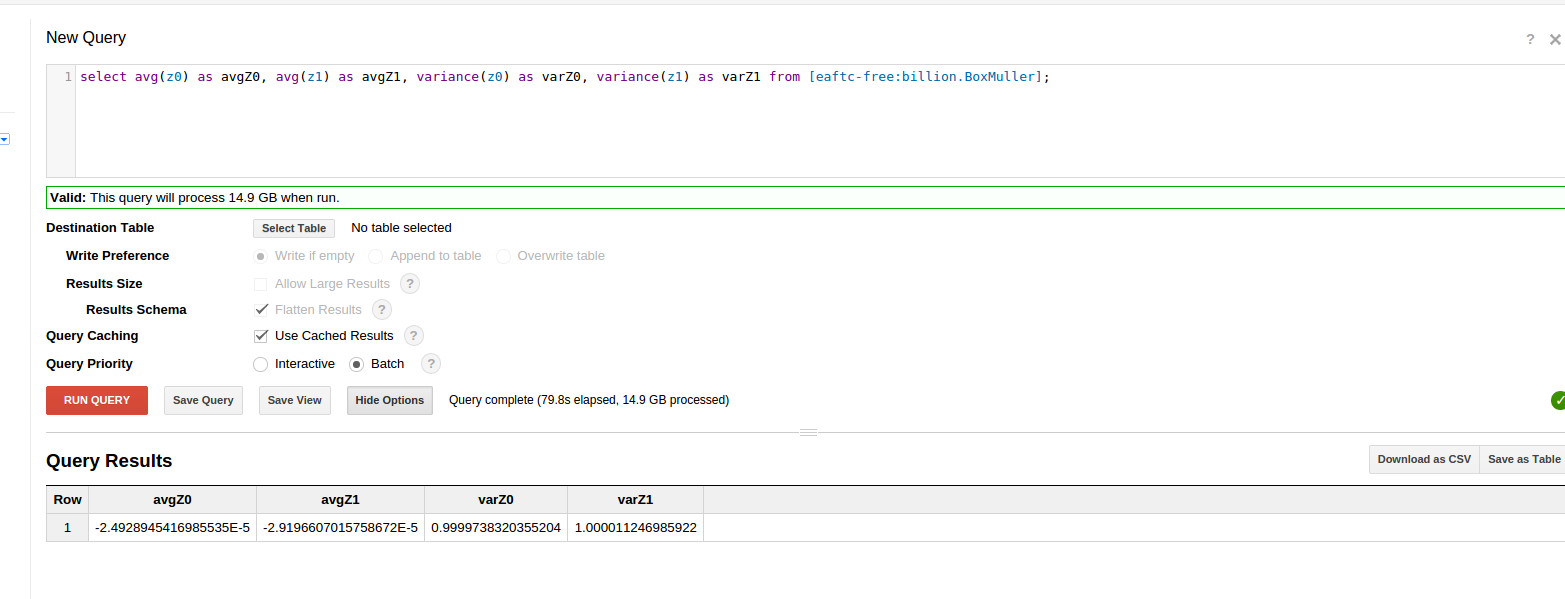# Examining the normal tail: That infamous sixth sigma

By six sigma here we are referring to the Normal distribution, not the Six Sigma managerial process and training organizations, although the goals of the latter are marketed through the mathematical properties of the former.
In the normal distribution `z0 >= 6.0` occurs with a probability of about 1 in a billion.
We can use Google BigQuery to see how many of these occurred in our billion normal values.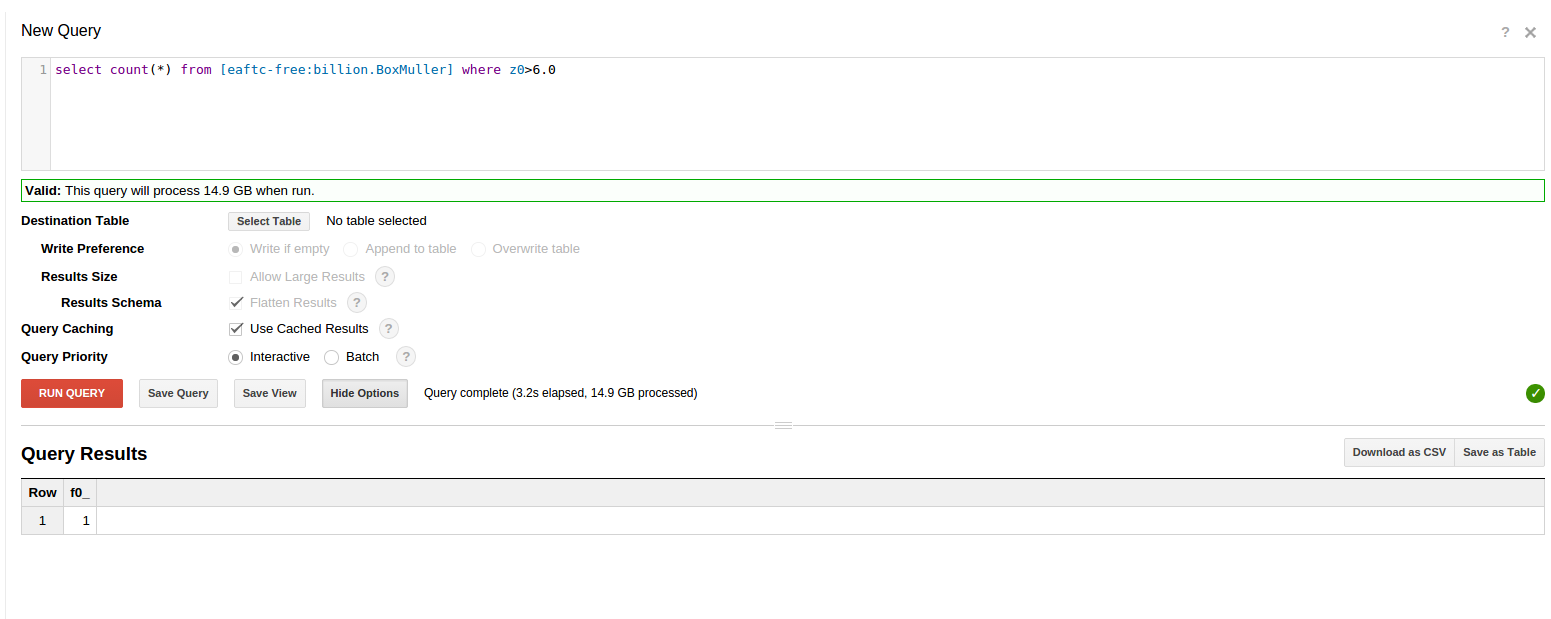and we can find the row of the Box Muller view with this value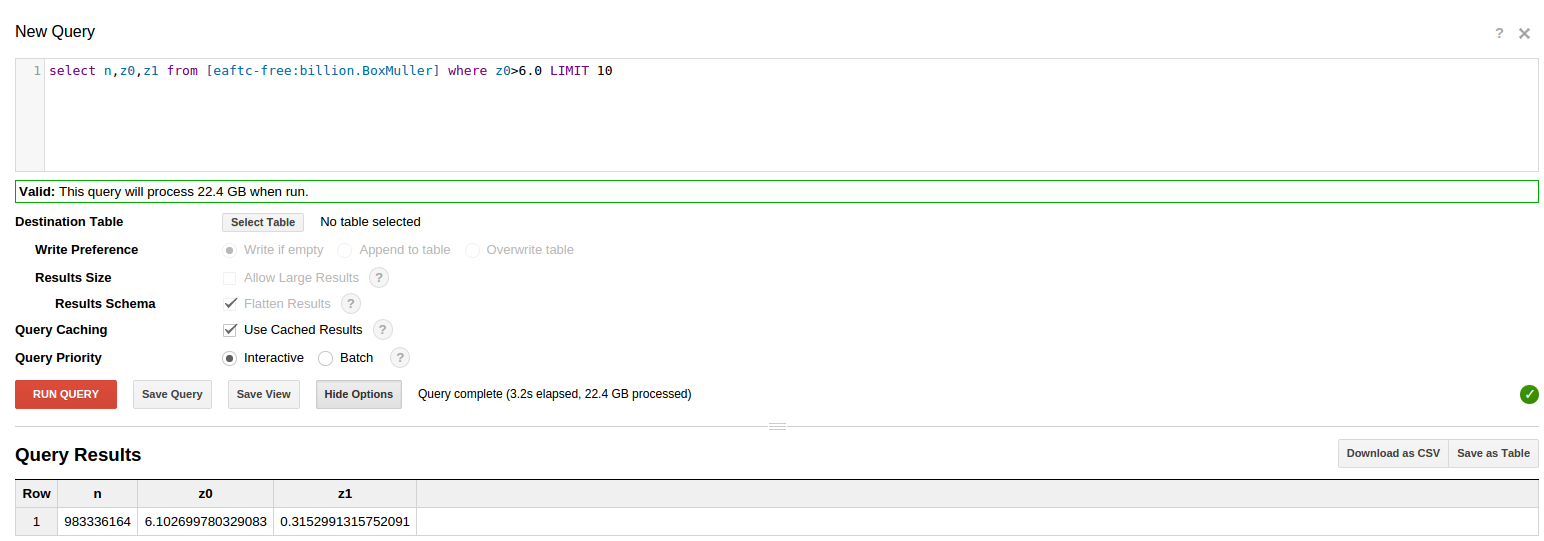# Five sigma

Exceeding five sigma should give us about 573 events per billion for a two sided (+ or -) deviation, or about 286 if we just look at one half… let’s look: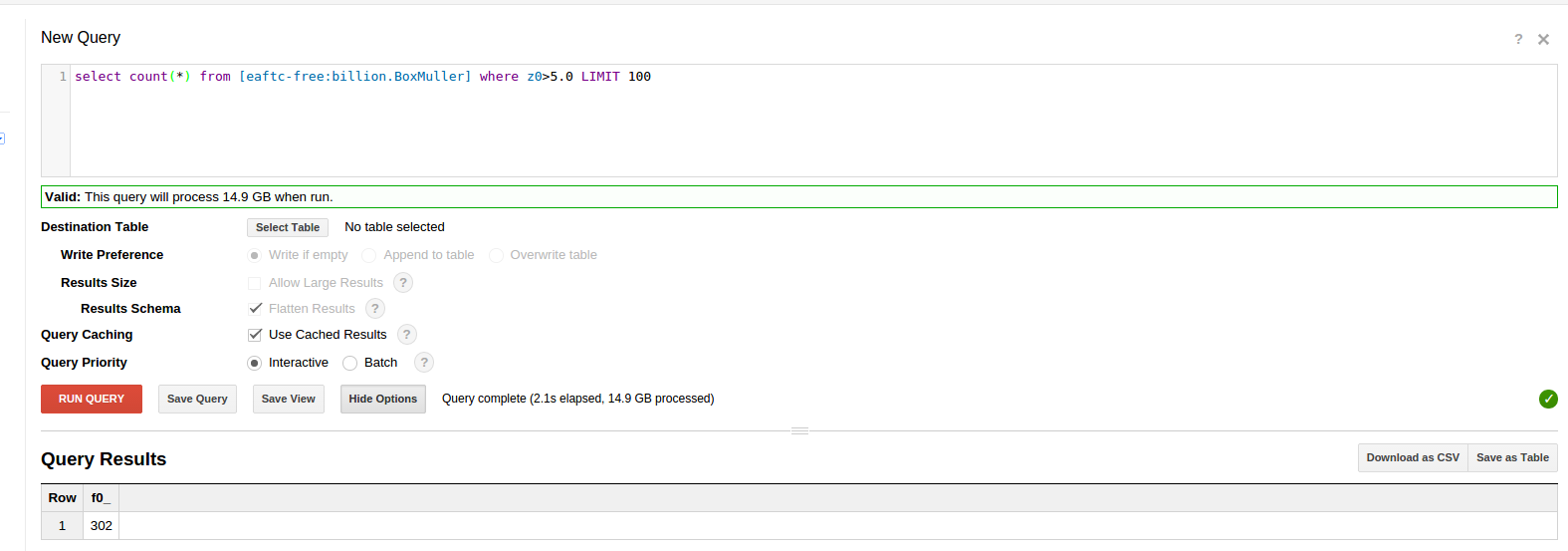We find 302 in the positive, or right tail.
Is this too many? No.
From The Law of Rare Events the standard deviation in the number of rare events is the square root of the expected number or, for 286 expected events, +/- 17 events.
302 is within one sigma of the expected value and thus not a statistically significant difference.

# Opportunity for Future work

Now that we have a way to produce standard random normal variables, it should be possible to build random walks as cumulative sums. Then, from a random walk, one can do Monte Carlo stock options pricing.
According to Stack Overflow, Google BigQuery supports cumulative sums
Further exploration of random walks is left to the reader or a future article.

# Conclusions

With some care and the lessons from this article, Google BigQuery can, in its current form, function as an interesting, massively parallel scientific calculator and simulation platform.
It might be even better if `rand()` were a little more well-behaved. Although this article did not consider whether the random number generator passes statistical quality tests or standards for random generation, even if the rand function failed such a tests it would be a fairly simple matter to upload a fairly large table of properly generated random numbers...
We don’t know whether Google wants to be in the simulation generation space, or only the data analysis space. This will probably be more clear as Google BigQuery develops, or if Google develops additional tools or products that can leverage their parallel processing capabilities and help users avoid the steep learning curves that otherwise exist for setting up and using most modern parallel technologies.